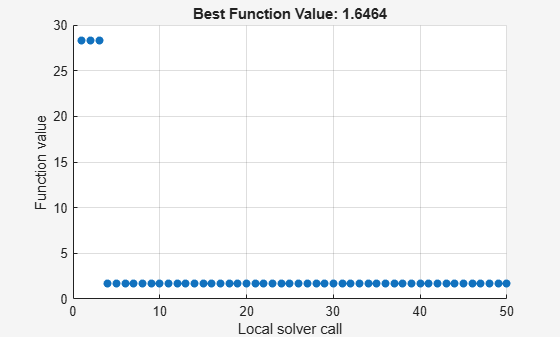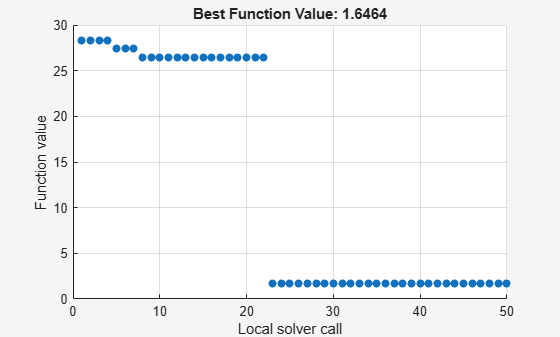Documentation

### This is machine translation

Mouseover text to see original. Click the button below to return to the English version of the page.

To view all translated materials including this page, select Country from the country navigator on the bottom of this page.

## MultiStart Using `lsqcurvefit` or `lsqnonlin`

This example shows how to fit a function to data using `lsqcurvefit` together with `MultiStart`. The end of the example shows the same solution using `lsqnonlin`.

Many fitting problems have multiple local solutions. `MultiStart` can help find the global solution, meaning the best fit. This example first uses `lsqcurvefit` because of its convenient syntax.

The model is

`$y=a+b{x}_{1}\mathrm{sin}\left(c{x}_{2}+d\right),$`

where the input data is $x=\left({x}_{1},{x}_{2}\right)$, and the parameters $a$, $b$, $c$, and $d$ are the unknown model coefficients.

### Step 1. Create the objective function.

Write an anonymous function that takes a data matrix `xdata` with `N` rows and two columns, and returns a response vector with `N` rows. The function also takes a coefficient matrix `p`, corresponding to the coefficient vector $\left(a,b,c,d\right)$.

`fitfcn = @(p,xdata)p(1) + p(2)*xdata(:,1).*sin(p(3)*xdata(:,2)+p(4));`

### Step 2. Create the training data.

Create 200 data points and responses. Use the values $a=-3,b=1/4,c=1/2,d=1$. Include random noise in the response.

```rng default % For reproducibility N = 200; % Number of data points preal = [-3,1/4,1/2,1]; % Real coefficients xdata = 5*rand(N,2); % Data points ydata = fitfcn(preal,xdata) + 0.1*randn(N,1); % Response data with noise```

### Step 3. Set bounds and initial point.

Set bounds for `lsqcurvefit`. There is no reason for $d$ to exceed $\pi$ in absolute value, because the sine function takes values in its full range over any interval of width $2\pi$. Assume that the coefficient $c$ must be smaller than 20 in absolute value, because allowing a high frequency can cause unstable responses or inaccurate convergence.

```lb = [-Inf,-Inf,-20,-pi]; ub = [Inf,Inf,20,pi];```

Set the initial point arbitrarily to (5,5,5,0).

```p0 = 5*ones(1,4); % Arbitrary initial point p0(4) = 0; % Ensure the initial point satisfies the bounds```

### Step 4. Find the best local fit.

Fit the parameters to the data, starting at `p0`.

`[xfitted,errorfitted] = lsqcurvefit(fitfcn,p0,xdata,ydata,lb,ub)`
```Local minimum possible. lsqcurvefit stopped because the final change in the sum of squares relative to its initial value is less than the value of the function tolerance. ```
```xfitted = 1×4 -2.6149 -0.0238 6.0191 -1.6998 ```
```errorfitted = 28.2524 ```

`lsqcurvefit` finds a local solution that is not particularly close to the model parameter values (–3,1/4,1/2,1).

### Step 5. Set up the problem for `MultiStart`.

Create a problem structure so `MultiStart` can solve the same problem.

```problem = createOptimProblem('lsqcurvefit','x0',p0,'objective',fitfcn,... 'lb',lb,'ub',ub,'xdata',xdata,'ydata',ydata);```

### Step 6. Find a global solution.

Solve the fitting problem using `MultiStart` with 50 iterations. Plot the smallest error as the number of `MultiStart` iterations.

```ms = MultiStart('PlotFcns',@gsplotbestf); [xmulti,errormulti] = run(ms,problem,50)``````MultiStart completed the runs from all start points. All 50 local solver runs converged with a positive local solver exit flag. ```
```xmulti = 1×4 -2.9852 -0.2472 -0.4968 -1.0438 ```
```errormulti = 1.6464 ```

`MultiStart` finds a global solution near the parameter values (–3,–1/4,–1/2,–1). (This is equivalent to a solution near `preal` = (–3,1/4,1/2,1), because changing the sign of all the coefficients except the first gives the same numerical values of `fitfcn`.) The norm of the residual error decreases from about 28 to about 1.6, a decrease of more than a factor of 10.

### Formulate Problem for `lsqnonlin`

For an alternative approach, use `lsqnonlin` as the fitting function. In this case, use the difference between predicted values and actual data values as the objective function.

```fitfcn2 = @(p)fitfcn(p,xdata)-ydata; [xlsqnonlin,errorlsqnonlin] = lsqnonlin(fitfcn2,p0,lb,ub)```
```Local minimum possible. lsqnonlin stopped because the final change in the sum of squares relative to its initial value is less than the value of the function tolerance. ```
```xlsqnonlin = 1×4 -2.6149 -0.0238 6.0191 -1.6998 ```
```errorlsqnonlin = 28.2524 ```

Starting from the same initial point `p0`, `lsqnonlin` finds the same relatively poor solution as `lsqcurvefit`.

Run `MultiStart` using `lsqnonlin` as the local solver.

```problem2 = createOptimProblem('lsqnonlin','x0',p0,'objective',fitfcn2,... 'lb',lb,'ub',ub'); [xmultinonlin,errormultinonlin] = run(ms,problem2,50)``````MultiStart completed the runs from all start points. All 50 local solver runs converged with a positive local solver exit flag. ```
```xmultinonlin = 1×4 -2.9852 -0.2472 -0.4968 -1.0438 ```
```errormultinonlin = 1.6464 ```

Again, `MultiStart` finds a much better solution than the local solver alone.

Watch now